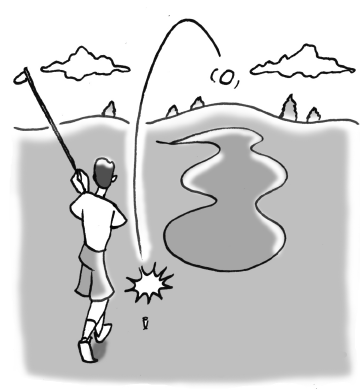### Home > AC > Chapter 10 > Lesson 10.1.4 > Problem10-40

10-40.To avoid a sand trap, a golfer hits a ball so that its height is represented by the equation h = −16t2 + 80t, where h is the height measured in feet and t is the time measured in seconds.

1. When does the ball land on the ground?

Substitute $0$ for $h$ to find how long it takes for the ball to land on the ground. $0=−16t^2+80t$

Solve by factoring.

$t=5\ \text{or}\ 0$

$t=0$ represents when the ball was on the ground before it started flight; therefore, it took $5$ seconds for it to land after its flight.

1. What is the maximum height of the ball during its flight?

Assume that the ball would be highest exactly halfway through its flight.
Knowing this information, substitute half of the total time it took the ball to land for $t$ and solve for $h$.